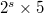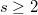﻿

### Primes modulo which almost all Fermat numbers are primitive roots

#### Abstract

A prime$p$ is called elite, or anti-elite, when all but finitely many      Fermat numbers are quadratic nonresidues or residues, respectively, modulo$p$. It is known that if the multiplicative order of 2 modulo$p$ is of the form$2^s\times 5$, where$s\geq 2$, then the prime$p$ is either elite or anti-elite. Modulo elite primes of this kind, we describe some criteria by which all sufficiently large Fermat numbers be primitive roots, or all nonprimitive roots.

DOI Code: 10.1285/i15900932v30n1p133

Keywords:
elite primes; Fermat numbers

Classification: 11A07, 11A41

Full Text: PDF